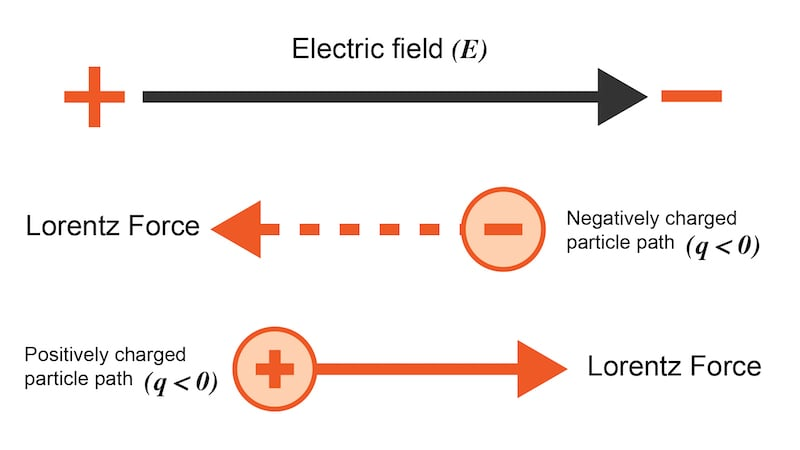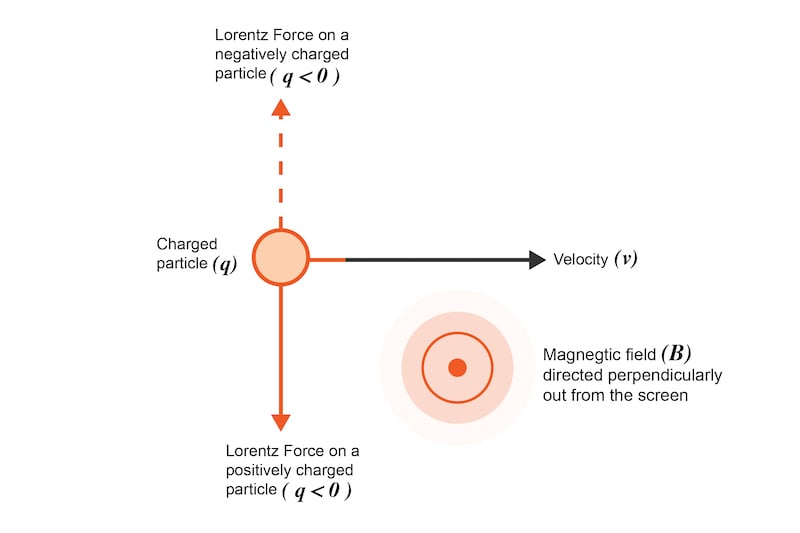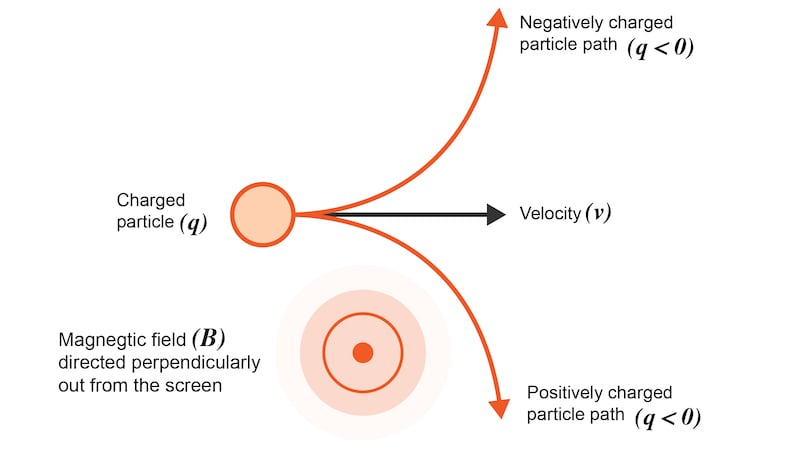# Lorentz Force Calculator

## This calculator will determine the combined Lorentz force exerted on a charged particle moving through both an electric field and a magnetic field.

### Lorentz Force Calculator

Enter any 5 of the 6 variables and the calculator will provide the missing value.

In this Lorentz force calculator, enter any five of the six variables and this calculator will provide the value for the missing parameter. The six variables in the Lorentz formula are the:

• Lorentz force
• Particle charge
• Particle velocity
• Electric field strength
• Magnetic field strength
• Relative angle of the velocity vector to the magnetic field vector

### Lorentz Force Definition—What is Lorentz Force?

The Lorentz force is the combined force exerted on a particle with charge q moving with velocity v through both a magnetic field B and an electric field E. Let's first examine the effects of the electric and magnetic fields independently.

### Lorentz Force Electric Field Component

The electric field imparts a force on the charged particle that is independent of the velocity vector of the particle. The direction of the force is a function of the polarity of the charged particle. A positively charged particle will be accelerated in the same linear orientation as the electric field, whereas a negatively charged particle will be accelerated in a direction opposite to the electric field. These effects can be seen in Figure 1.### The Magnetic Field and Lorentz Force

The magnetic field imparts a force on the charged particle that is a function of both the polarity of the charge and the velocity of the particle. This is a cross-product such that the force is perpendicular to both the velocity vector and the magnetic field vector (Figure 2).##### Figure 2. The Lorentz force acting on a charged particle moving through a magnetic field

Due to this, the particle will curve perpendicularly to both the instantaneous velocity vector and the magnetic field, as shown in Figure 3.### How to Calculate Lorenz Force—the Lorentz Force Equation

To determine the total force exerted on a charged particle moving through both a magnetic field and an electric field, we can sum the forces exerted by both individually. This is expressed using the following Lorentz force equation:

$$F_l = qE + q v \times B$$

##### Equation 1. Lorentz force equation

Where:

• Fl = the Lorentz force expressed in Newtons
• q = the total charge of the particle expressed in coulombs
• E = The electric field expressed in V/m (or equivalently N/C)
• v = The velocity of the charged particle expressed in m/s
• B = The magnetic field expressed in teslas

As can be seen from the equation, the Lorentz force is the sum of the force exhibited by the electric field on the particle (qE) and the force exhibited by the magnetic field on the particle (qv X B). If you need to find the force exhibited by only one field, the other field’s strength can be set to 0.

The contribution of the magnetic field to the total Lorentz force is a cross product, meaning that it is dependent on the angle of the magnetic field relative to the particle’s velocity. Based on this, we can further simplify the Lorentz force formula to the following:

$$F_l = qE + q v B \sin(\theta)$$

##### Equation 2. Simplified Lorentz force equation

Where:

• $$\theta$$ = The angle between the particle’s velocity vector and the magnetic field vector, expressed in radians

For this calculator, the angle is limited to:

$$-\frac{\pi}{2} \le \theta \le \frac{\pi}{2}$$

Or, equivalently, if the angle is expressed in degrees:

$$-90\le \theta \le 90$$

### Lorentz Force Applications

Understanding this force is important in analyzing or designing applications such as motors, sensors, speakers, and imaging. Other applications of the Lorentz force include:

• Cyclotrons and other particle accelerators
• Bubble chamber for tracking the trajectories of charged particles.